## Sidebar: Description of FIR Filters

FIR filters are often used because they are simple and easy to understand . Diagrams of the operation of an FIR filter are shown in Figure S1. An FIR filter works by multiplying an array of the most recent n data samples by an array of constants (called the tap coefficients), and summing the elements of the resulting array. (This operation is commonly called a dot product.) The filter then inputs another sample of data (which causes the oldest piece of data to be thrown away) and repeats the process.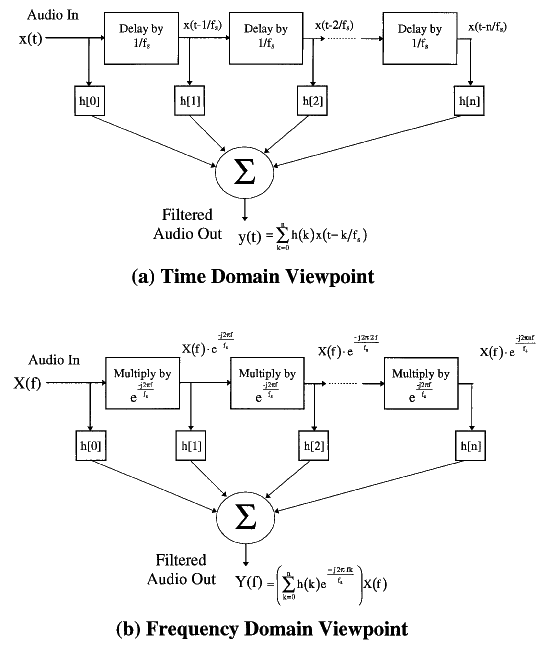Sidebar Fig - (a) FIR Filter operation in the time domain. Given the h[k]'s, we are implementing an FIR filter as shown in the diagram. (b) FIR Filter operation in the frequency domain. This diagram shows the frequency response of the filter in part a. Notice that the tap coefficients (h[k]'s) are simply the Fourier series coefficients of the frequency response.

The interesting part of designing FIR filters is translating the desired frequency response into filter tap coefficients. As can be seen from Figure S1a, the equation for the output of an FIR filter in the time domain is: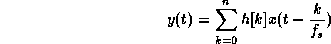(Eq. S1)

where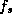is the sampling frequency and k is the filter tap number. Since a delay of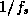in time corresponds to a multiplication by a complex exponential, the corresponding equation in the frequency domain (from Figure S1b) is:(Eq. S2a)

where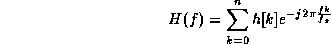(Eq. S2b)

is the frequency response of the FIR filter. It can be seen that h[k], the filter tap coefficients, are precisely the Fourier series coefficients of H(f) which is periodic with period. Therefore the tap coefficients may be calculated from the following equation: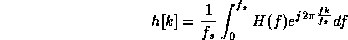(Eq. S3)

An additional option for improving the performance of the filters, is to apply a window function to the filter tap coefficients . Coefficients affecting the higher frequencies are scaled down to reduce the ripple in the stop band. This can be done as follows: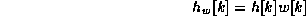(Eq. S4)

where w[k] are the window scale factors, h[k] are the original tap coefficients, and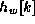are the windowed tap coefficients. The resulting coefficients may now be used in place of the original ones. An unwanted side effect of windowing, however, is the sacrifice of a sharp roll-off at the cutoff frequency.

So, the design process is to pick the desired frequency response, H(f), and then calculate the tap coefficients (Eq. S3). The actual frequency response will only approximate the desired response because the number of filter taps is finite. Optionally, a window function may then be applied to the filter tap coefficients. The final step is to plot the actual frequency response, H(f), using Eq. S2b to make sure the resulting response is acceptable. Lousy responses can be tweaked using different windowing schemes.